# On the Behavior of 2d Transformations in Internet Explorer, Part 2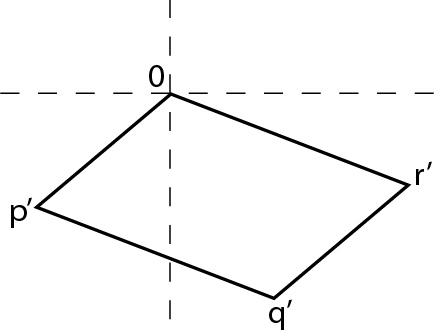Unsurprisingly, the last post ran overlong. I’ll endeavor to stick to code and equations wherever possible, as they are generally faster for me to write and you to parse. Amateur-hour Illustrator work takes a little longer.

All previous provisos still apply.

### Implementation

If you’ve waded through that enormity of a post, you now know everything you need to get transformations working in Internet Explorer. To review:

1. Keep an internal augmented matrix to represent the current transformation. Manipulate it through some combination of programmatic access and the parsing of CSS-like transform functions.
2. Apply the linear portion of the affine transformation (the top 2×2 entries of the matrix) to the element through IE’s Matrix Filter
3. Use “auto expand” as the SizingMethod so the element can venture beyond its original confines.
4. Determine the shift that IE has automatically applied through its “bounding box behavior.”
5. Translate the element by increasing its left and top attributes by the translation from the internal matrix, less the shift found in step (4).
6. Work around minor problems like the limits of integer translations and problems due to altering layout via repositioning.

I’ll run through each of these items in turn, sharing implementation details and some of the interesting behavior I ran across in testing. If you’re the impatient type (or the “I don’t care” type), source is here.

### 1: Internal Representation

The biggest win from this approach comes from the standard model/view split of the representation of the transformation and the actual DOM style of the element. For one, this means that all transformation DOM access is one way: nothing needs to be read. This also allows transformation-changing methods to alter only the matrix representation, which can happen in superfast JavaScript-land, leaving a single style-setting DOM access (or as few as possible) until just before the execution context is yielded.

My module uses GWT’s scheduleFinally() mechanism to automate this process, accumulating transformation changes as they occur; each change only flags the need for a style update and doesn’t actually make one. Usually this means that the actual layout of an element is only updated once per animation frame—in a method named commitTransform()—and only if its transformation was actually changed. Combined with the fact that authors don’t have to intervene in this process (though it’s possible to do so), this is about as good as it gets.

For the update, I’ve given up using internal 4×4 matrices on platforms (IE, Firefox) with no 3d transform support. I originally chose to use them out of hope for the future and to maybe do some tricksy things with projections, but the tradeoff—for now—isn’t worth it. Obviously the 4×4 implementation will remain, lying in wait, but for the present IE and Firefox will default to 3×3 matrices and the > 50% savings in matrix multiplications. It’s no DOM access optimization, but in complicated transformations, the ops add up quickly.

(It’s worth noting that Chrome and Safari use a totally different 4×4 matrix implementation, which is built around their native WebKitCSSMatrix. Due to its design (instances are immutable) memory use is quite a bit higher, but the tradeoff is that all matrix ops take place in native code. I’m planning on moving Firefox to its version of MozCSSMatrix (or even MozSVGMatrix) when it’s available.)

A note for anyone doing this in straight JavaScript: please use a matrix library optimized for 2d or 3d transforms. I’ve noticed that the Sylvester library is widely used. While it is a great library, my understanding is that it was written for general matrix support, so you’ll get things like loop-based matrix multiplication, generalized inversion methods, and a lot of utilities that you’ll never use. When you only have 9 (or 16) matrix entries, a lot of these things can be massively sped up through special-case algorithms. Check out Vladimir Vukićević’s mjs library for something specifically intended for this purpose.

Finally, I currently only support procedural access to manipulating an element’s transformation, but this means that any transform set in a stylesheet will be clobbered. See Transformie for a good example of the parsing of an existing (and changing) list of transformation functions.

### 2: Applying the Linear Transformation

Interestingly, I’ve found that setting the Matrix Filter’s properties individually (and numerically) ends up causing significantly longer layout delays than setting the whole thing as a string. That is, this:

var matFilter = target.filters['DXImageTransform.Microsoft.Matrix']; matFilter.M11 = a; matFilter.M21 = b; matFilter.M12 = c; matFilter.M22 = d;

tends to somehow be much slower than the more naive

var filt = 'progid:DXImageTransform.Microsoft.Matrix(M11=' + a; filt += ', M21=' + b; filt += ', M12=' + c; filt += ', M22=' + d + ')'; target.style['filter'] = filt;

Obviously the access method is different, so perhaps the matrix-filter object has been deprecated internally and it all goes through string parsing anyway. Or perhaps every set variable triggers an internal DOM change rather than using one lazy write. I’m not sure of the reason, but I’ve found that the first approach takes almost twice as long as the second, so I no longer use it. I’d be interested if anyone has other data points on the subject.

Unlike the current CSS3 implementations, IE will happily accept scientific notation in either case; don’t worry about number formatting through toFixed() or the like.

### 3: “auto expand”

I’m still convinced that whoever wrote SizingMethod didn’t like other humans and/or didn’t use their own browser.

### 4: The Bounding-Box Shift, Take 1

As a reminder, here again is the shifting behavior of an element under a pure rotation in Internet Explorer: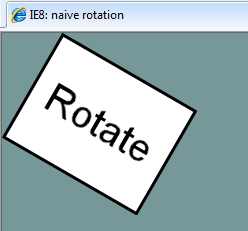In the previous post, I assumed that we knew the dimensions of the transformed element’s axis-aligned bounding box when calculating IE’s bonus translation. By far the easiest way to find this information is to first set the linear portion of the transformation to the element’s matrix filter, then ask the element for its current offsetWidth and offsetHeight. IE faithfully updates these values to give the distance between the extremities of the transformed element in each dimension.

As determined last time, the x-shift we need to remove is just half the original width, subtracted from half the bounding width. The same pattern applies vertically.

// original layout var x = target.offsetLeft; var y = target.offsetTop; var w = target.offsetWidth; var h = target.offsetHeight;   ...   setInterval(function(){ // matrix filter applied ...   // find bounding box dimensions // IE has updated these values based on transform set above var wb = target.offsetWidth; var hb = target.offsetHeight;   // determine how far origin has shifted var sx = (wb - w) / 2; var sy = (hb - h) / 2;   // translation, corrected for origin shift // rounding helps, but doesn't eliminate, integer jittering target.style.left = Math.round(x + e - sx) + 'px'; target.style.top = Math.round(y + f - sy) + 'px';   }, 30);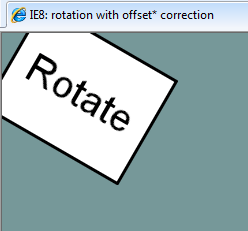Well done, theory. This works, and since it works, this is essentially how I left my original implementation. I knew this approach was inherently slow, but (as I wrote before) I didn’t need to animate transformations so I didn’t worry about it.

The reason this method is slow is hopefully obvious. To describe its actions in a different way, it writes to an element’s style, reads computed values from its updated style—forcing at least a limited reflow—then writes to it again, all within the same execution context. Certain DOM disaster.

I ran head-on into this problem when I started to fling little boxes across the screen. Even with a full 2d physics library simulating the boxes, geometric transformations being calculated per box, and each box’s element being positioned absolutely to keep it out of the document flow, these four lines of code took up a full 40% of execution time.

A different approach is needed.

### 4a. Better Box Bounds

The straightforward way to calculate the size of the minimum axis-aligned bounding box of a transformed rectangle is to apply the linear transformation to each corner of the rectangle in turn. From the resulting points, find the minimum and maximum x- and y-coordinates, subtract, et voilà.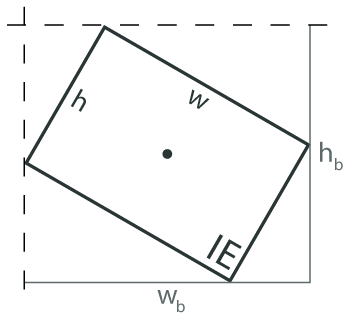But that’s boring, redundant, and the nuns used to tell me that extra conditionals are the path to wickedness. Let’s see if we can do better.

First, we don’t care about the bounding box’s actual coordinates, we just need its width and height.

Second, because we only care about the width and height, we can translate the element and its bounding box in any way we wish. A translation affects all points in the same way, so the distance between two points is invariant under a translation. If we translate the element in such a way that its top left corner is moved to the origin, we will only have to transform 3 points, since linear transformations leave the origin in place.

The specific translation needed is irrelevant; conceptually, the element is now just a conveniently positioned rectangle with width w and height h.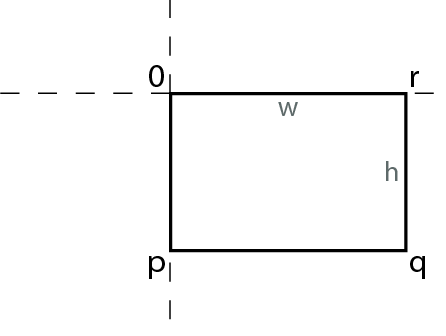The non-origin corners of the rectangle have also been simplified. Their positions:$\mathbf{p} = \begin{bmatrix}0 \\ h \end{bmatrix}$$\mathbf{q} = \begin{bmatrix}w \\ h \end{bmatrix}$$\mathbf{r} = \begin{bmatrix}w \\ 0 \end{bmatrix}$

(We’re still in screen coordinates, so positive y points down).

Now let’s look at the same rectangle, under some transformation T. Since we’re still in step (4), T is just a linear transformation (and so is represented by a 2×2 matrix). Here is an image of the rectangle under one possible TIn general, if$\mathbf{T}=\begin{bmatrix}a & c \\ b & d \end{bmatrix}$

then the coordinates of the transformed corners are$\mathbf{p}'=\mathbf{T}\mathbf{p}=\begin{bmatrix}a & c \\ b & d \end{bmatrix}\begin{bmatrix}0 \\ h \end{bmatrix}= \begin{bmatrix}ch \\ dh \end{bmatrix}$$\mathbf{q}'=\mathbf{T}\mathbf{q}=\begin{bmatrix}a & c \\ b & d \end{bmatrix} \begin{bmatrix}w \\ h \end{bmatrix}=\begin{bmatrix} aw + ch \\ bw + dh \end{bmatrix}$$\mathbf{r}'=\mathbf{T}\mathbf{r}=\begin{bmatrix}a & c \\ b & d \end{bmatrix} \begin{bmatrix}w \\ 0 \end{bmatrix}= \begin{bmatrix}aw \\ bw \end{bmatrix}$

For the example transformed rectangle, now with a projected bounding box: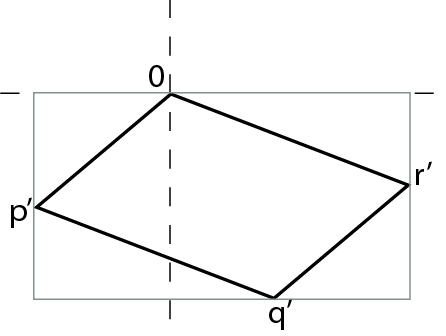it should be clear that in this case the width of the bounding box is$w_b=r_x'-p_x'$

By substitution,$w_b=aw-ch$

Incidentally, because ch is negative (see image), this is equivalent to$w_b=aw+|ch|$

(hint, wink, nudge, etc).

The height of this bounding box is$h_b=q_y'-0=bw+dh$

We need to determine the difference between the greatest and the least of the x-coordinates and the y-coordinates, for all transformations. Rather than trying to enumerate all the possible geometric situations, it’s easier to consider sign combinations. Beginning with the x-coordinates, we have four values: 0, ch, aw, and (aw + ch). a and c can be any real number, while w and h will always be non-negative.

• Case 1: aw and ch are positive. 0 is clearly the minimum x-value and (awch) is both the maximum and the width of the bounding box.
• Case 2: aw and ch are negative. 0 is now the maximum and (awch) the minimum, but the bounding box’s width—the distance from the maximum to the minimum x-value—is still |(aw + ch)|.
• Case 3: aw and ch have opposite signs. 0 cannot be a max or a min, as it is bounded by these values. Likewise, (aw + ch) will also be bounded by the two. It does not matter which value is positive and which negative, since the subtraction of the negative value will yield the addition of its magnitude; the width is (|aw| + |ch|) either way.
• Finally, careful consideration of the cases where aw or ch is equal to 0 (always be careful with your 0s) will find that their outcomes fall under the results listed above.

Since w and h are always non-negative, we only have to worry about the signs of the entries in T. While half the time it is a waste of an abs() call, it is always safe to say that$w_b=|a|w+|c|h$

Therefore, if sx is the horizontal distance between the ideal origin and IE’s shifted origin, then, using our shift equation and substituting the new wb$s_x=\frac{1}{2}(w_b-w)=\frac{1}{2}(|a|w+|c|h-w)$$s_x=(|a|-1)\frac{w}{2} +|c|\frac{h}{2}$

Similarly,$s_y=|b|\frac{w}{2} + (|d|-1)\frac{h}{2}$

### All Together Now

You have a 2d affine transformation, represented by an augmented matrix of the form$\mathbf{T}=\begin{bmatrix}a & c & e\\ b & d & f \end{bmatrix}$

To transform an element by this matrix in some future, standards-compliant browser, the following is sufficient:

target.style.transform = "matrix(a, b, c, d, e, f)";

Today, just substitute the vendor-extended version for “transform,” and add <length> units to e and f for Firefox (which doesn’t really make any sense, but for now: fine).

To do the same in Internet Explorer, here is our assembled JavaScript routine. In spite of some minor math optimizations, it does end up being fairly straightforward. Feel free to substitute your favorite IE string concatenation method.

// set linear transformation via Matrix Filter var filt = 'progid:DXImageTransform.Microsoft.Matrix('; filt += 'M11=' + a; filt += ', M21=' + b; filt += ', M12=' + c; filt += ', M22=' + d; filt += ', SizingMethod="auto expand")'; target.style['filter'] = filt;   // assuming a-d are local variables // and halfW and halfH are initialized properly   // horizontal shift a = Math.abs(a); // or go ternary c = Math.abs(c); var sx = (a - 1)*halfW + c*halfH;   // vertical shift b = Math.abs(b); d = Math.abs(d); var sy = b*halfW + (d - 1)*halfH;   // translation, corrected for origin shift // rounding helps--but doesn't eliminate--integer jittering target.style.left = Math.round(x + e - sx) + 'px'; target.style.top = Math.round(y + f - sy) + 'px';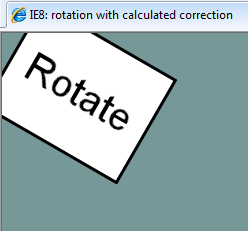The shift correction is not exactly self-documenting code, but it is kind of pretty. The dimensions of the bounding box are computed—not queried—from the current linear transformation and used to correct the position of the element’s origin. commitTransform() now treats the DOM as write-only.

From some empirical testing, I’ve found that for a pure rotation this method will vary from the slow version by no more than a pixel in each dimension, and usually by much less. This error seems to grow at a quarter of the scale factor: for example, an element scaled by a factor of 20 will be at most 5 pixels off from where the slow method would place it. Based on the performance improvement, outlined below, I find this to be an acceptable trade-off (and without further testing, I’m not sure I would conclusively say that the slow method is more correct, anyway).

And there you have it; a mostly foolproof, efficient transformation method for Internet Explorer. If you can express your desired 2d affine transform in terms of a 2×3 matrix, and it’s not hard to do so, this code will take care of the rest.

### The GWT Code

I originally wasn’t going to include this—everything one needs to implement IE support is found above—but I think it’s interesting to see what the GWT compiler does with the code. This snippet comes straight from the IE permutation of my test program, but has been complied using the “PRETTY” compiler option so it is somewhat comprehensible. I’ve inserted some extra comments for additional explanation.

Keep in mind that, even though this is the less-minified “pretty” output, it is meant to be an end-product and not an intermediate one. As such, syntax normally considered to be unfriendly (like the comma operator) is fine in this context.

// this$static is object scope function$commitTransform(this$static){ var finalTransform, m11, m12, m21, m22, tarStyle, xAdj, yAdj; // init dimensions if this is the first time transforming element if (!this$static.elementInitialized) { if ($isOrHasChild(($clinit_22() , $doc.body), this$static.target)) { $initElementLayout(this$static); } else { return; } }   // only hint that compiler removed unused origin-changing code finalTransform = this$static.transform; // horizontal shift (using 'a' and 'c') m11 = finalTransform.m11; m11 = m11 < 0?-m11:m11; m12 = finalTransform.m12; m12 = m12 < 0?-m12:m12; xAdj = (1 - m11) * this$static.halfOrigWidth - m12 * this$static.halfOrigHeight; // vertical shift (using 'b' and 'd') m21 = finalTransform.m21; m21 = m21 < 0?-m21:m21; m22 = finalTransform.m22; m22 = m22 < 0?-m22:m22; yAdj = -m21 * this$static.halfOrigWidth + (1 - m22) * this$static.halfOrigHeight; // translation from matrix ('e' and 'f') xAdj += finalTransform.m13; yAdj += finalTransform.m23; tarStyle = this$static.target.style;   // I use Array.join, but doesn't make a huge difference tarStyle['filter'] = (filterArray = finalTransform.m11 , filterArray = finalTransform.m12 , filterArray = finalTransform.m21 , filterArray = finalTransform.m22 , filterArray.join(''));   tarStyle['left'] = this$static.originalLeft + xAdj + 'px'; tarStyle['top'] = this$static.originalTop + yAdj + 'px'; }

The GWT compiler doesn’t really have a chance to work its magic here; the java code was purposefully written to be straightforward, so the output is close to what was input. What’s interesting is actually what you don’t see. There’s barely a hint that the compiler was able to remove the code for handling setOrigin(), which is nicely inlined if it is used. There is also no trace of the completely different code that is used in Firefox, or Chrome, or Opera, etc.

Incidentally, this little gem takes care of everything found above in the Webkit permutation:

function $commitTransform(this$static){ this$static.target.style.WebkitTransform = this$static.transform; }

I’m trying to think of the appropriate cliche to sum up poor old IE’s situation—something about bailing water or running in place—but I just don’t have the heart.

### DOM is the animation-killer

It can be difficult to get exact numbers for code that affects reflow and repainting. Beyond the usual “measuring JavaScript with JavaScript” problems, other style-changing code can have a huge impact on execution time, and the non-deterministic order in which they all may interleave can have disproportionate effects. As a result, I’ve tried to rely on numbers from my stress-test program, which in some ways resembles a real web application. The results come from the built-in IE8 profiler, so specific values shouldn’t be treated as absolute.

In addition to the changes already mentioned, I’ve made a few other efficiency improvements. I restructured the initialization of internal “scratch” matrices to avoid GWT’s clinit()s (Firefox shows a 75% reduction in null method calls). Combined with the switch to 3×3 matrices, optimization of the bounding box routine, and other small changes, I managed to cut the execution time of the write-only version of commitTransform() by about 50%.

For all my bluster about numerical and algebraic improvements, though, this amounts to about a tenth of a millisecond, depending on how it’s measured. This is a big savings and the faster commitTransform() can yield sooner and let other code run more often. But it is nothing compared to the elimination of reading DOM attributes.

In the example files, averaged over 20,000 calls, the offsetWidth/Height transformation method takes 2.5ms. On the other hand, the final transformation method claims to take 0.0ms, but my division finds an average of .03ms per call (though I could imagine an uncertainty approaching .1ms). But that’s just a toy example.

As I mentioned above, in an already intensive app, commitTransform() on 40 elements just 10 times per second was using around 40% of total execution time on a maxed-out processor. The new version drops this below 4%. To emphasize the point: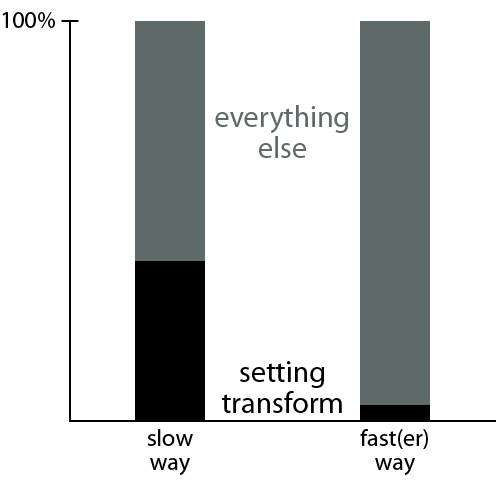Keep in mind that “setting transform” isn’t calculating the transform, it isn’t changing it, it isn’t making a new one; it is only setting the transform style from an existing matrix. Note also that “everything else” is everything else, including the time the app spends responding to user input, which is its only reason for existing in the first place.

Now, transforming and positioning elements correctly is important, but I think the second bar better reflects the time that should be devoted to just setting the transform style on 40 elements.

Two take-aways:

1. USE THE SECOND METHOD. Unless you’re certain that the original bounds of the elements have changed (and there are event listeners for that sort of thing), cache the bounds and calculate the bounding box.
2. If you aren’t paying attention to the way you access the DOM, your application will be slow. This is certainly nowhere near an original statement, but it deserves to be repeated yet again. Firebug, SpeedTracer, and IE’s surprisingly handy profiler will help.

Internet Explorer’s commitTransform() is now on par with Firefox’s (IE actually profiles quite a bit faster, but with way too many variables to control, I’ll stick with “on par”). Limiting agents are now elsewhere.

### Future Work

Overall, it’s been interesting to get back into this code. As I wrote at the beginning, this module didn’t get the full attention it needed, so the code isn’t exactly a paragon of software design (or good code formatting). Part of the problem was that encapsulating transformations in a way that matches the behavior of CSS3 transforms—the forward-thinking approach—is made difficult by the particulars of the IE implementation.

For instance, I still think a transform shouldn’t be tied to a particular element; it is, after all, just a style (even if I tend to use transformed elements as logical entities). But to do transformations in IE quickly, the original size of an element can only be read and cached once, so a particular transform is bound to a particular element and the abstraction is lost. This can be worked around (and to an extent already is in the Transforms/TransformedElement split), but currently there is a conceptual gap between the exposed interface and what it gives access to. It’s fine, but it’s not elegant.

For now, however, the IE code is working well and speedily (according to the browser’s ability). In the months since I started this project, I’ve developed a better sense for the GWT UI idioms (and some thoughts on integrating with UiBinder), so a rewrite is definitely overdue. However, writing this post gave me an idea for yet another way one might abuse the transform system, so I’ll probably put off any redesign until I have a better idea of all use cases. For the time being, feel free to check out and use the current module and provide any feedback on how it could better suit your needs.

All the source code is here: gwt-ns project page.

### Examples, Again

This entry was posted in Uncategorized and tagged , , , , , , , . Bookmark the permalink.

### 3 Responses to On the Behavior of 2d Transformations in Internet Explorer, Part 2

1.Bundyo says:

Looks like the key for the Matrix filter to work in IE6/7 is that SizingMethod needs to be the last in the filter properties, otherwise the CSS parser breaks (or whatever parses the filters – the rest of the CSS doesn’t seem affected) and every filter after the first is ignored.

•Dave says:

Wow. Bundyo, thank you! I’ve been tearing my hair out for 2 days now, trying to figure out why IE6 & 7 were ignoring everything after the first call (and not applying the sizing method correctly: using a rotate transform, the elements were scaled up as well). Shifting the sizingMethod to the last filter property auto-magically fixed it.